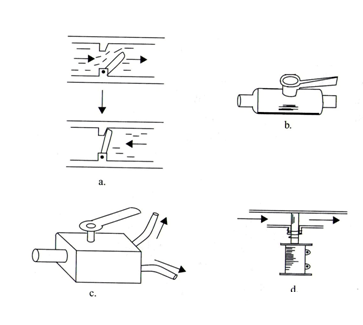### DatasheetsN° of component

## Using the Hydraulic Pressure (MEC216E)

Hydraulic machines can be used to obtain nechanical advantage. The principle is in the use of pistons but basically we can understand how they work starting from simple tubes as shown by figure 1.

Mechanical advantage is obtained in the configuration shown by the figure and can be calculated by the next formula:

Formulas

a) Force gain

## F1/F2 = S1/S2

Where:

F1 is the force applied to the small piston

F2 is the force applied to the large piston

S1 is the are of the small piston

S2 is the are of the large piston

b) displacement

## F1/F2 = d2/d1

Where:

F1 is the force applied to the small piston

F2 is the force applied to the large piston

d1 is the displacemente of the small piston

d2 is the displacement of the large piston

## TMA = F2/F1 = d1/d2 = S2/S1

Where:

TMA is the Theoritical Mechanical Advantage

F1 is the force applied to the small piston

F2 is the force applied to the large piston

d1 is the displacemente of the small piston

d2 is the displacement of the large piston

S1 is the are of the small piston

S2 is the are of the large piston

## 3.4 - Valves

Valves are devices used to allow a fluid to flow only in one direction.

Figure 2 shows the typical construction of some valves.(a) automatic

(b) manual valve

(c) derivation valve

(d) solenoid valve

## Practical Hydraulic Devices

Solenoids combined with pistons and valves can result in devices with applications in mechatronics. Figure 3 shows some of combinations with applications in practical designs.

(a) Solenoid valve

(b) Solenoid actuator

(c) Hydraulic switch

(d) Electric pump

(e) Rotary to linear hydraulic converter

(f) hydraulic motor